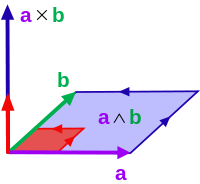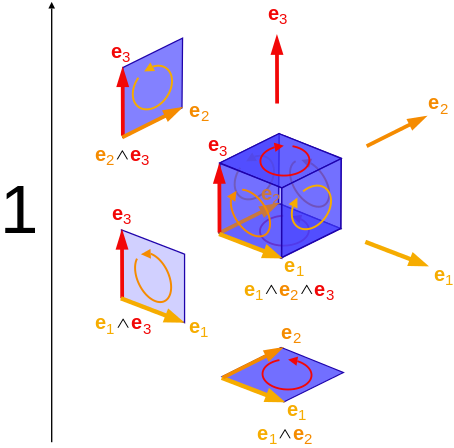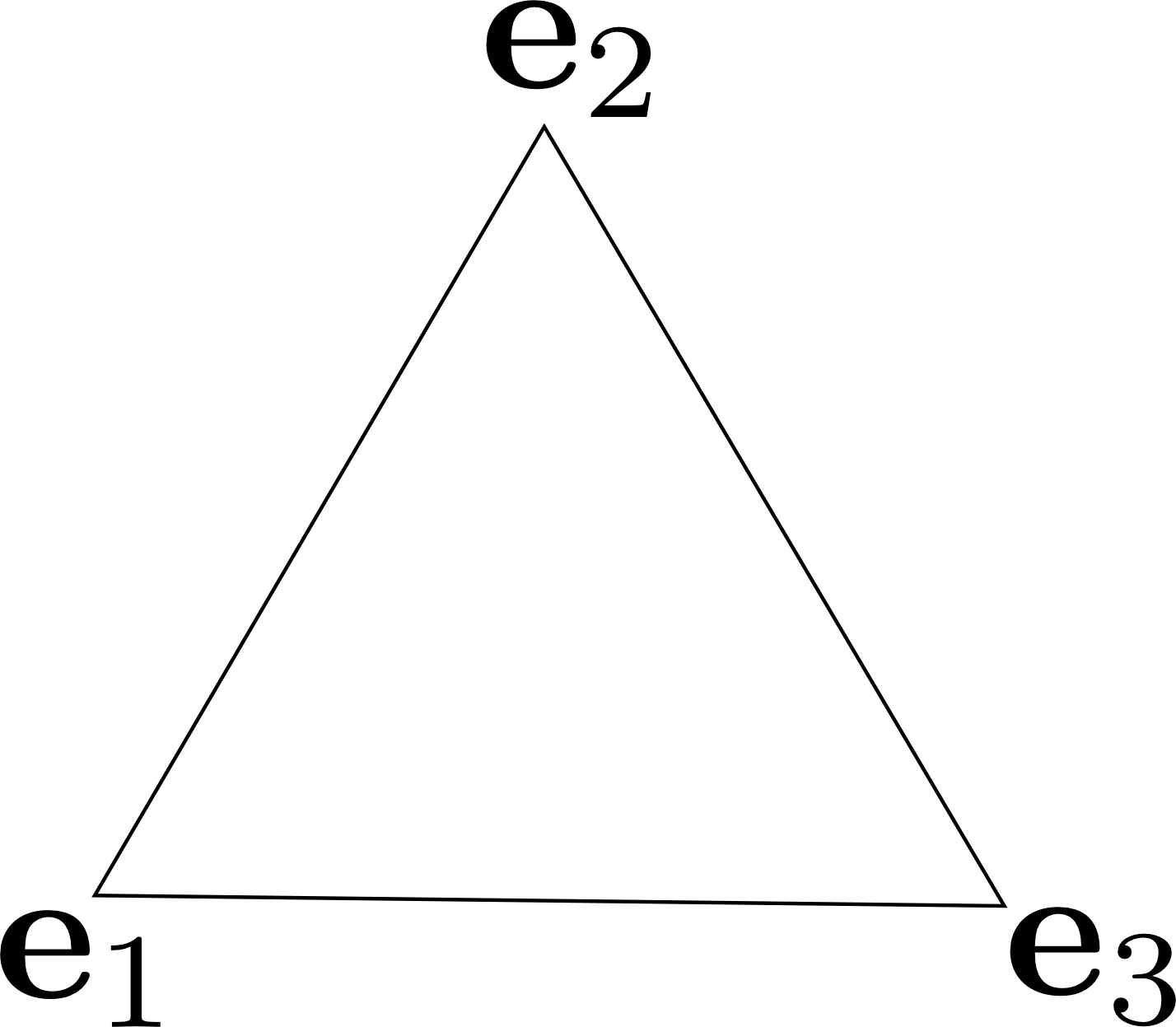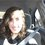# The Strange Lands of Geometrized Quantum Mechanics

Prologue

This note is a one-way ticket to the fantastical lands of geometry and quantum mechanics. As your concierge, I will be showing you around these curious lands. As we partake in our adventure, I will equip you with the necessary tools to navigate uncharted territory in the quantum realm using a powerful tool developed by William Clifford. Your objective: explore. But be warned: the roads that lie ahead are so potently enigmatic they defeated the great Einstien.

Fasten your socks. We are about to go on one wild ride.

Introduction

Quantum mechanics (QM) is a notoriously counter-intuitive topic. The physics of the very small, for the better half of the century, has remained enigmatic and inaccessible to the general public. The mathematical underpinning of the subject is often insurmountable for the curious reader -- this is where I come in. With nifty magic, I reduce the quantum realm into delicious bite-sized pieces of knowledge! Eat as many as you like, but prepare yourself for a brain blow.

We will explore the central theme that separates QM from classical physics. I aim to break the subject down to its irreducible building blocks, leaving you with the rudimentary tools required to further explore the bewildering world of quantum mechanics.

The Classical Realm

In grade school, we were taught the Real numbers $\mathbb{R}$ are commutative: $ab = ba$. In other words, we are free to multiply from either the left or the right; the order does not matter.

In classical physics, a and b take the form of an observable. That is, a and b are physical quantities we measure in the real world. An observable can take the value of any Real number $\mathbb{R}$ with units that correspond to the physical quantity being measured. For the purposes of this note, we take a and b as the position x and momentum p observables. Commutativity between position and momentum always holds true:

$xp = px$

Thus, the order the position x and momentum p are measured does not matter -- the outcome of the measurement will always be the same.

The Quantum Realm

In High school, we were taught that the $n \times m$ matrices with entries belonging to the Real numbers $\mathbb{R}$, denoted as $\mathbb{R}^{n \times m}$, are in general not commutative: $AB \neq BA$. In other words, the order the matrix A and matrix B are multiplied does matter.

In Quantum mechanics, a and b do not take the form of an observable. Instead, they take the form of an operator. We think of an operator $\hat{A}$ as a measurement on a physical system that returns the observable a. In general, operators in QM do not commute: $\hat{A}\hat{B} \neq \hat{B}\hat{A}$. That is to say, the order the observables a and b are measured with the operators $\hat{A}$ and $\hat{B}$ does matter.

Similar to classical mechanics, in QM the operator $\hat{A}$ takes the form of the position operator $\hat{x}$ and the operator $\hat{B}$ takes the form of the momentum operator $\hat{p}$. In the quantum realm, $x$ is the outcome of measurement $\hat{x}$ and $p$ is the outcome of measurement $\hat{p}$.

Classically, physical objects have an unambiguous position $x$ and momentum $p$, before, during, and after any measurement. Quantum mechanically, physical objects have neither a well-defined position $x$ nor momentum $p$ before any measurement $\hat{x}$ or measurement $\hat{p}$ occurs. Mathematically, this counter-intuitive property is captured by the so-called canonical commutation relation:

$[\hat{x}, \hat{p}] := \hat{x}\hat{p} - \hat{p}\hat{x} = i\hbar$

The above equation reads as follows: The difference between measuring the position $x$ before the momentum $p$ and the position $x$ after the momentum $p$ is the imaginary constant $i$ multiplied by the quantum of action $\hbar$. Here, the quantum of action (commonly referred to as Planck's reduced constant) $\hbar$ has units $joules \cdot seconds$ and can be thought of as the minimum amount of energy imparted on a physical object through the action of any measurement.

Heisenberg’s uncertainty principle,

$\sigma_{x}\sigma_{p} \geq |\dfrac{1}{2i}<[\hat{x}, \hat{p}]>| = \dfrac{\hbar}{2}$

which states that neither the position $x$ nor the momentum $p$ can be measured with complete precision is a fundamental consequence of the canonical commutation relation.

The Realm of the Exterior Algebra

A problem of great concern to many 19th century mathematicians was how to most effectively represent rotations in 3-dimensional space. The first serious attempt to solve this problem was offered by Hamilton, who introduced the quaternions. During Hamilton's development of his quaternionic algebra, Grassmann was formulating his own algebra. Grassman constructed his algebra through the introduction of the exterior product. The exterior product of two vectors $\textbf{a}$ and $\textbf{b}$ (denoted as $\textbf{a} \wedge \textbf{b}$), has two primary properties: associativity,

$\textbf{a} \wedge (\textbf{b} \wedge \textbf{c}) = (\textbf{a} \wedge \textbf{b}) \wedge \textbf{c}$

and anticommutativity

$\textbf{a} \wedge \textbf{b} = -\textbf{b} \wedge \textbf{a}$

In contrast, the inner product of two vectors, $\textbf{a}$ and $\textbf{b}$ (denoted as $\textbf{a} \cdot \textbf{b}$), is commutative,

$\textbf{a} \cdot \textbf{b} = \textbf{b} \cdot \textbf{a}$

The exterior product of two vectors $\textbf{a}$ and $\textbf{b}$ constitutes a new quantity called a bivector. The bivector $\textbf{a} \wedge \textbf{b}$ is the oriented area swept out by the vectors $\textbf{a}$ and $\textbf{b}$:

$\textbf{a} \wedge \textbf{b} = \text{det}[\textbf{a} \quad \textbf{b}]\textbf{e}_{1} \wedge \textbf{e}_{2}$where $\textbf{e}_{1}$ and $\textbf{e}_{2}$ are the basis vectors of $\mathbb{R}^{2}$. Likewise, the trivector $\textbf{a} \wedge \textbf{b} \wedge \textbf{c}$ is the oriented volume swept out by the vectors $\textbf{a}$, $\textbf{b}$, and $\textbf{c}$:

$\textbf{a} \wedge \textbf{b} \wedge \textbf{c} = \text{det}[\textbf{a} \quad \textbf{b} \quad \textbf{c}]\textbf{e}_{1} \wedge \textbf{e}_{2} \wedge \textbf{e}_{3}$

where $\textbf{e}_{1}$, $\textbf{e}_{2}$, and $\textbf{e}_{3}$ are the basis vectors of $\mathbb{R}^{3}$.The Enchanting Realm of Geometric Algebra

Clifford united the algebras of Hamilton and Grassman by defining the so-called geometric product:

$\textbf{ab} = \textbf{a} \cdot \textbf{b} + \textbf{a} \wedge \textbf{b}$

In 2-dimensions, the orthonormal basis vectors $\textbf{e}_{1}$ and $\textbf{e}_{2}$ satisfy

$\textbf{e}_{1}^{2} = \textbf{e}_{2}^{2} = 1$ and $\quad \textbf{e}_{1} \cdot \textbf{e}_{2} = 0$

Thus, the geometric product of the basis vectors is a bivector:

$\textbf{e}_{1}\textbf{e}_{2} = \textbf{e}_{1} \cdot \textbf{e}_{2} + \textbf{e}_{1} \wedge \textbf{e}_{2} = \textbf{e}_{1} \wedge \textbf{e}_{2} = -\textbf{e}_{2} \wedge \textbf{e}_{1} = -\textbf{e}_{2}\textbf{e}_{1}$

Now this is where the real magic happens. If we square the above bivector we get

$(\textbf{e}_{1}\textbf{e}_{2})^{2} = \textbf{e}_{1}\textbf{e}_{2}\textbf{e}_{1}\textbf{e}_{2} = -\textbf{e}_{1}\textbf{e}_{1}\textbf{e}_{2}\textbf{e}_{2} = -(\textbf{e}_{1})^{2}(\textbf{e}_{2})^{2} = -1$

A real geometric object that squares to $-1$? Welcome to the rabbit hole, Alice.

What's more surprising: It isn't the only quantity that squares to $-1$, in fact generalizing the geometric algebra for $N$-dimensional Euclidean space, yields a multivector for any dimension $N$ that squares to $-1$.

WARNING: Uncharted Territory

From the geometric algebra we can define a geometric commutator:

$[\textbf{e}_{i}, \textbf{e}_{j}] = \textbf{i}_{2}\alpha$

Here, $\textbf{i}_{2} = \textbf{e}_{i}\textbf{e}_{j}$ and $\alpha = \pm 2$.

Inserting the geometric commutator (for Euclidean 3-space) in the Heisenberg uncertainty relation yields:

$\sigma_{\textbf{e}_{i}}\sigma_{\textbf{e}_{j}} \geq |\dfrac{1}{2i}<[\textbf{e}_{i}, \textbf{e}_{j}]>| = \begin{pmatrix} 0 & 1 & 1 \\ 1 & 0 & 1 \\ 1 & 1 & 0 \end{pmatrix}$

The above is an adjacency matrix for a complete graph on 3 vertices.So, what in the world does this mean?Note by Maya Benowitz
3 years, 5 months ago

This discussion board is a place to discuss our Daily Challenges and the math and science related to those challenges. Explanations are more than just a solution — they should explain the steps and thinking strategies that you used to obtain the solution. Comments should further the discussion of math and science.

When posting on Brilliant:

• Use the emojis to react to an explanation, whether you're congratulating a job well done , or just really confused .
• Ask specific questions about the challenge or the steps in somebody's explanation. Well-posed questions can add a lot to the discussion, but posting "I don't understand!" doesn't help anyone.
• Try to contribute something new to the discussion, whether it is an extension, generalization or other idea related to the challenge.

MarkdownAppears as
*italics* or _italics_ italics
**bold** or __bold__ bold
- bulleted- list
• bulleted
• list
1. numbered2. list
1. numbered
2. list
Note: you must add a full line of space before and after lists for them to show up correctly
paragraph 1paragraph 2

paragraph 1

paragraph 2

[example link](https://brilliant.org)example link
> This is a quote
This is a quote
    # I indented these lines
# 4 spaces, and now they show
# up as a code block.

print "hello world"
# I indented these lines
# 4 spaces, and now they show
# up as a code block.

print "hello world"
MathAppears as
Remember to wrap math in $$ ... $$ or $ ... $ to ensure proper formatting.
2 \times 3 $2 \times 3$
2^{34} $2^{34}$
a_{i-1} $a_{i-1}$
\frac{2}{3} $\frac{2}{3}$
\sqrt{2} $\sqrt{2}$
\sum_{i=1}^3 $\sum_{i=1}^3$
\sin \theta $\sin \theta$
\boxed{123} $\boxed{123}$